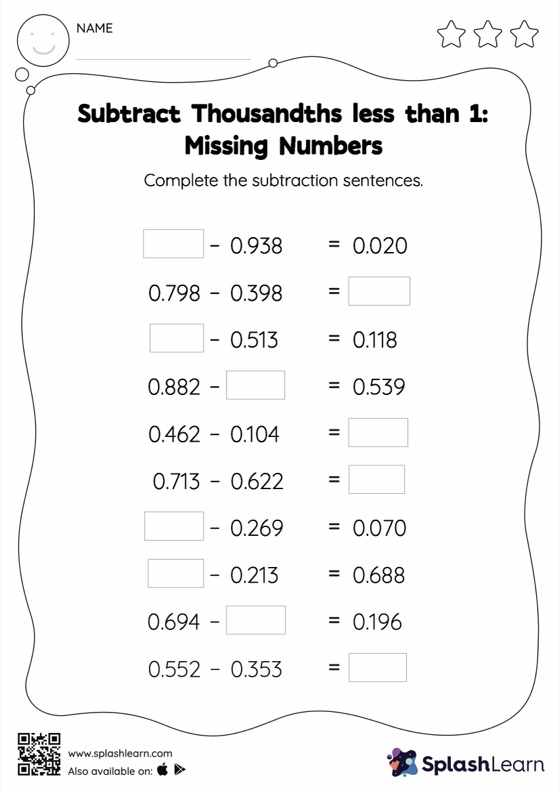# Subtract Thousandths less than 1: Missing Numbers Worksheet

Home > Subtract Thousandths less than 1: Missing NumbersDoes your child know how to subtract thousandths less than 1? Invite them to practice this concept here. In this worksheet, students subtract decimals by aligning the decimal points and using zero as a placeholder. They then use the relationship between addition and subtraction to find the missing number in the subtract thousandths less than 1 worksheet. In each problem, the numbers are laid out in the horizontal format. Students should try to use different strategies involving composing and decomposing numbers to solve these problems. This will help them develop flexibility and fluency.